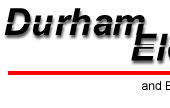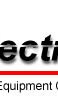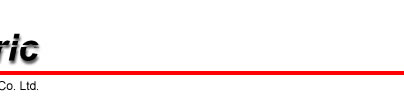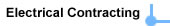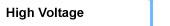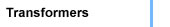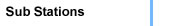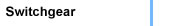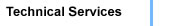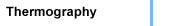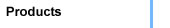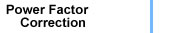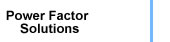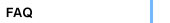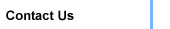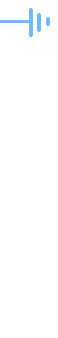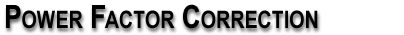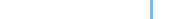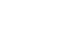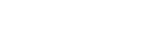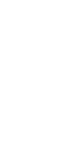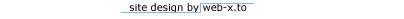page 2

POWER FACTOR (PF) is the ratio of real power to reactive power and is expressed as a percentage.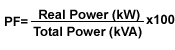Simply expressed, electrical systems are made up of three types of loads; resistors, inductors and capacitors. Resistive loads include incandescent lighting, baseboard heaters and ovens. Inductive loads such as electric motors, ballasts, transformers and arc welders require an electromagnetic field to operate. Inductive loads have both real and reactive power components. The power factor of an inductive load is referred to as lagging or less than 100% based upon the power factor ratio. Capacitors also require a component of reactive power to operate. However capacitors and inductors have an opposite effect on reactive power. The power factor of a capacitor is leading and therefore counteracts the effect of reactive power used by an inductive load.

>> Power Factor Solutions